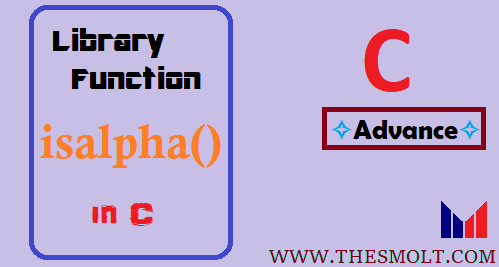# How to use Isalpha Function in C and Replicate C isalpha()

## Isalpha Function in C

The isalpha() is a library function in the C language for checks whether your entered string is an alphabet or not. Let’s learn Isalpha Function in C through a Program.

## Use of isalpha in C

```#include <stdio.h>

#include <ctype.h>

int ft_str_is_alpha(char *str)

{
int index = 0;
int result = 0;

while(str[index] != '\0')

{

if(isalpha(str[index])!=0)

{
result = 1;
}
else
{
result = 0;
break;

}

index++;

}

// printf("\nr: %d", result);
return(result);

}

int main()

{
char c[] = "helloWaaa";
printf("%d\n",ft_str_is_alpha(c));
printf("%s",c);

}```
Output
1 helloWaaa
Bit changed used built-in function, u can do the same with your logic.

## Replicate isalpha in C

2nd Run Replicate isalpha()

#include <stdio.h>

#include <ctype.h>

int ft_str_is_alpha(char *str)

{

int index = 0;

int result = 0;

while(str[index] != ‘\0′)

{

if((str[index]>=’a’ && str[index] <=’z’) || (str[index]>=’A’ && str[index] <=’Z’) )

{

result = 1;

}

else

{

result = 0; break;

}

index++;

} // printf(“\nr: %d”, result);

return(result);

}

int main()

qk[o

{

char c[] = “hello1Waaa”;

printf(“%d\n”,ft_str_is_alpha(c));

printf(“%s”,c);

}

## Isalpha in C

Output
0

helloWaaa

The moment you encounter a character other than the alphabet, like else part of ur condition, then you set

result =0;

and immediately break; the loop, and in the end when you return the result it will have 0.

if ur condition is always true like it has all alphabet,

then it will set result=1; and in the end, u will return result=1;

### C isalphaI hope you will understand about isalpha in C.

END

This site uses Akismet to reduce spam. Learn how your comment data is processed.